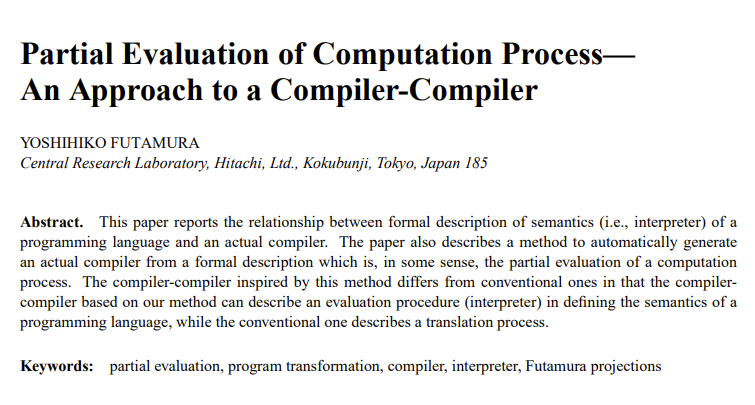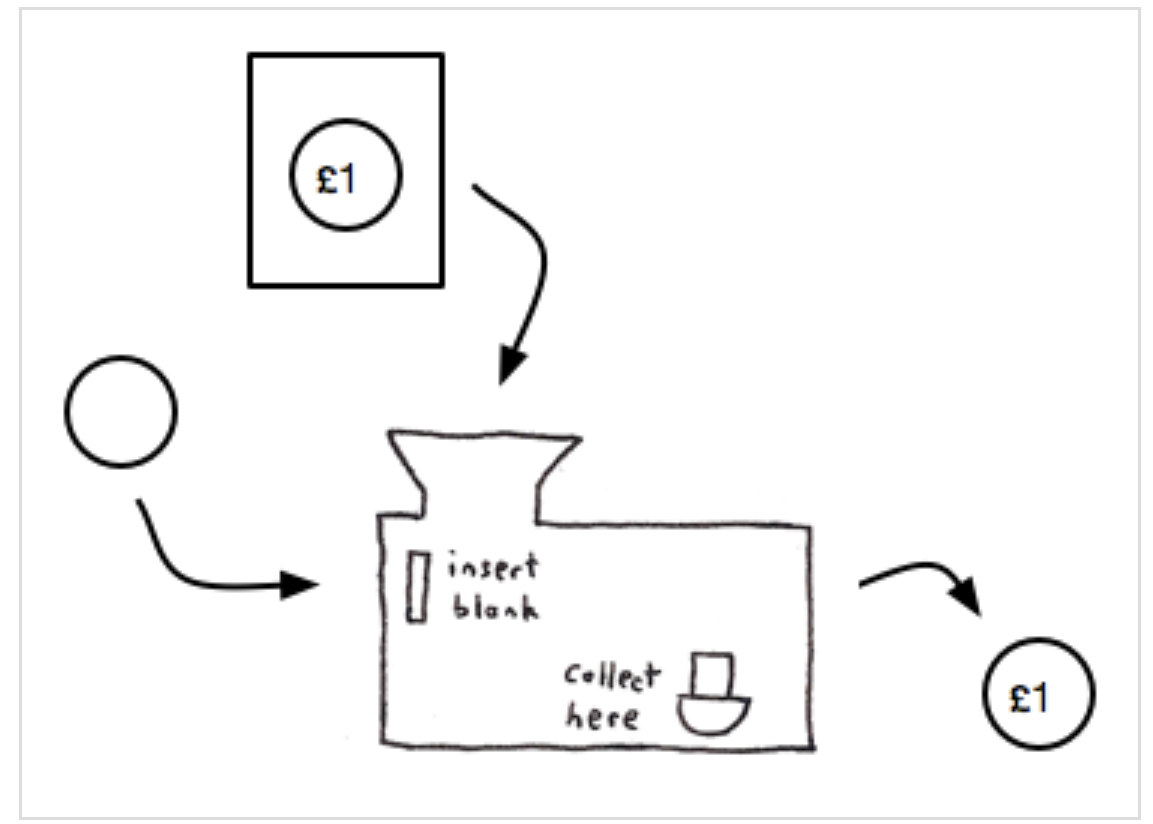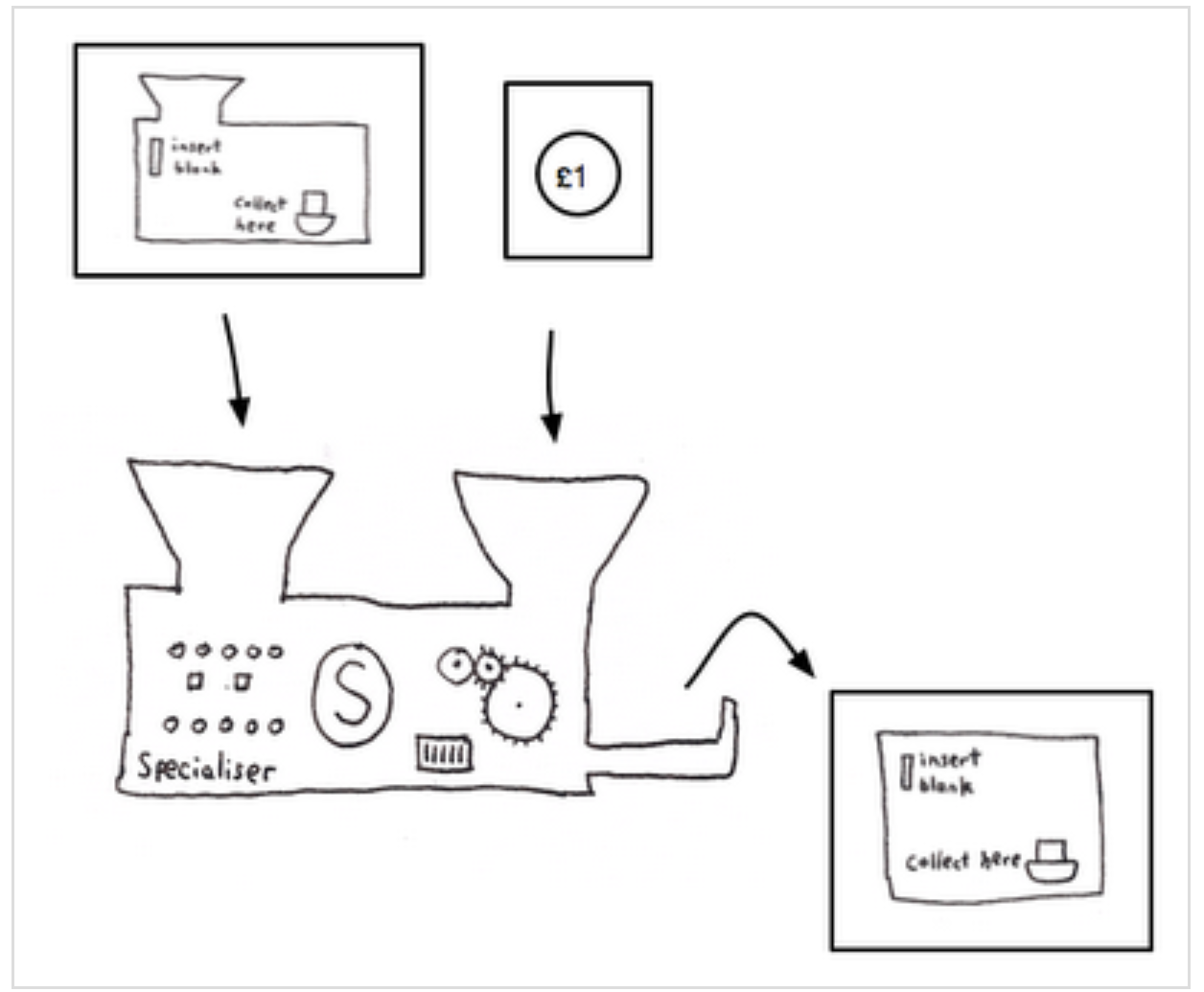Or optimizing a C code generator used in Windows, using

• Dependent Types
• Futamura Projections
• Denotational Semantics
• Normalization by Evaluation

The State of Play: March 2021

• The EverParse toolchain is deployed in the Windows build

• From about 5000 lines of spec, it produces about 25,000 lines of verified C code used in Hyper-V's Virtual Switch

• Representing about 2 years of work, hitting several Windows milestones

• Now part of Windows 11
• Backported to Windows Server 2019 etc.
• Since then

• Several new features, in support of additional scenarios requested by various networking teams

New features

• Stream Parsing

• Instead of only parsing from a contiguous array of bytes, EverParse can now be used to parse from any instantiation of a stream typeclass

• Useful for parsing in scatter/gather IO scenarios

• Or parsing huge formats, e.g., virtual hard disks etc.

• Parse trees

• More flexible support for parsing raw bytes into user-defined parse trees, using provably type-safe imperative parsing actions
• Error handling

• User provided callbacks can reconstruct a backtrace of a parsing failure

But, compilation times were slow

• Verifying and compiling 5000 lines of 3D to 25K lines of C takes about 5 minutes

• Not too bad on the face of it: 25K lines of verified C code in 5 mins
• Some of it can be parallelized, but even then, compiling the spec of a single protocol (RndisGuest) takes about 90 seconds

• Considering that a full build of Virtual Switch without EverParse itself takes ~8-10 mins, adding 5 mins to build time, or even 90s, is a source of pain

• We worked around it by adding hashes to the generated code, checking it in, to skip re-verifying files if the spec didn't change

This talk

How we improved compilation times in EverParse

• Overall sequential build time of EverParse in Virtual Switch is now 82s

• Still parallelizable, with the longest running task taking 17s

• 3.5x speedup on average and as much as 6x on larger programs

Using a bunch of fun PL techniques

The structure of an EverParse program

You write down a spec like this in a language called 3D:

struct OP {  UINT32 fst;  UINT32 snd { fst <= snd }; }

This spec induces 3 constructs in F* (lots of simplifications here)

1. A type of dependent pairs, representing F*'s view of this source type

let op = fst:UINT32 & snd:UINT32{fst <= snd}
2. A parser spec, which describes the layout of the type in an array of bytes

let parser t = b:bytes -> option (x:t & nat) { ... and some conditions here ... }
let parse_op : parser op = p_dep_pair p_u32 (fun fst ->
p_refine p_u32 (fun snd ->
if fst <= snd then p_ret (fst, snd)
else fail))
3. A validator in Low*, refining the parser spec, compilable to C

let validator (p:parser t) = input:array U8.t -> pos:U32.t -> len:U32.t -> ST errcode
(requires (* some preconditions, liveness of input etc. *))
(ensures (* the validator refines the parser spec p *))
let validate_op : validator parse_op = v_dep_pair v_u32 (fun fst ->
v_refine v_32 (fun snd ->
if fst <= snd then true
else false))
4. But it's sort of the same program 3 times!

Main idea

Let's parse the concrete syntax into one description of the program

• interpret it in three different ways (type, parser spec, validator)

• prove that those three interpretations are compatible once and for all

• and generate code from the validator interpretation

Should give us a roughly 3x speedup

Step 1: The denotation of 3D programs

• Semantically, every 3D program corresponds to this object in F*
type dtyp = { t:Type; p:parser t; v:validator p }
• A triple of {t; p; v} related to each other by typing.
• dtyp: Denotation of a 3D Type, or “defined type”
• Given a 3D program, our goal is to compute the dtyp corresponding to it

Step 2: Representing 3D programs

• A kind of higher-order abstract syntax, but mixing both shallow and deep embeddings

type prog =
| T_defined : dtyp -> prog //every type that is already defined can be used in a program

| T_pair : prog -> prog -> prog

| T_refine : d:dtyp -> (d.t -> bool) -> prog //refinements of defined types

| T_dep_pair : d:dtyp -> (d.t -> prog) -> prog //dependence on defined type

| T_case : b:bool -> (b==true -> prog) -> (b==false -> prog) -> prog

| ... //~19 cases

Step 3: Computing the denotation

let denote (pr:prog) : dtyp = { t=as_type pr; p=as_parser pr; v=as_validator pr}
where

let rec as_t : prog -> Type = function
| T_defined dt -> dt.t
| T_pair p1 p2 -> as_t p1 & as_t p2
| T_refine d f -> x:d.t{ f x }
| T_dep_pair dt p -> x:dt.t & as_t (p x)
| T_case b th el -> if b then th() else el()

let rec as_p : p:prog -> parser (as_t p) = function
| T_defined dt -> dt.p
| T_pair p1 p2 -> p_pair (as_p p1) (as_p p2)
| T_refine d f -> p_refine d.p f
| T_dep_pair dt p2 -> p_dep_pair dt.p (fun x -> as_p (p2 x))
| T_case b th el -> if b then as_p (th()) else as_p (el())

let rec as_v : p:prog -> validator (as_p p) = function
| T_defined dt -> dt.v
| T_pair p1 p2 -> v_pair (as_v p1) (as_v p2)
| T_refine d f -> v_refine d.v f
| T_dep_pair dt p2 -> v_dep_pair dt.v (fun x -> as_v (p2 x))
| T_case b th el -> if b then as_v (th()) else as_v (el())

Step 3: Computing the denotation

let denote (pr:prog) : dtyp = { t=as_type pr; p=as_parser pr; v=as_validator pr}
where

let rec as_t : prog -> Type = function
| T_defined dt -> dt.t
| T_pair p1 p2 -> as_t p1 & as_t p2
| T_refine d f -> x:d.t{ f x }
| T_dep_pair dt p -> x:dt.t & as_t (p x)
| T_case b th el -> if b then th() else el()

let rec as_p : p:prog -> parser (as_t p) = function
| T_defined dt -> dt.p
| T_pair p1 p2 -> p_pair (as_p p1) (as_p p2)
| T_refine d f -> p_refine d.p f
| T_dep_pair dt p2 -> p_dep_pair dt.p (fun x -> as_p (p2 x))
| T_case b th el -> if b then as_p (th()) else as_p (el())

let rec as_v : p:prog -> validator (as_p p) = function
| T_defined dt -> dt.v
| T_pair p1 p2 -> v_pair (as_v p1) (as_v p2)
| T_refine d f -> v_refine d.v f
| T_dep_pair dt p2 -> v_dep_pair dt.v (fun x -> as_v (p2 x))
| T_case b th el -> if b then as_v (th()) else as_v (el())
• as_t: An F* program computing a type by writing a recursive function over some data structure? No problem. It's dependent types!
• Dependently typed generic programming
• Same deal as giving a type to printf

Step 3: Computing the denotation

let denote (pr:prog) : dtyp = { t=as_type pr; p=as_parser pr; v=as_validator pr}
• That's it. That's our proof that every 3D program has a semantics as a validator
• In reality, it's about 1200 lines of code, handling many bells and whistles.

Ok, nice proof, but what's it useful for?

• Our function as_v is actually a little interpreter

• Given any p:prog it returns an imperative program (built from EverParse combinators) that can read an input array of bytes and parse it according to the format described by p.

• And, no way Windows is going to run an interpreter for data format programs in the middle of the kernel

Futamura ProjectionFutamura ProjectionFutamura Projection• Thanks to Ben Zorn who pointed me to this blog when I was telling him a while back about Futamura projections

First Futamura Projection

• Turn an interpreter into a compiler, by partially evaluating the interpreter with respect to particular inputFirst Futamura Projection

• Turn an interpreter into a compiler, by partially evaluating the interpreter with respect to particular inputSo, if only we could …

• Partially evaluate
as_v (T_pair dtyp_u32 dtyp_u32)
• We'd get an EverParse validator for a pair of U32s

• But, where to get a partially evaluator for F*?

• It already has one! All dependently typed languages do

Dependent types and definitional equality

• The central rule of a dependently typed language

• Terms are defintionally equal up to convertibility

• is equal to because they have the same normal form
• To implement this rule, a dependently typed language has to implement some machinery to compute with lambda terms

• So F*'s type system already knows how to reduce F* terms

• And that in the rule it means that the system has to be able to reduce terms with free variables

• i.e., is equal to

But, how to reduce a term efficiently?

• F* has a normalizer, based on a call-by-name abstract machine. But, it can be pretty slow if you get it to reduce large terms

• Instead, F* also has a call-by-value engine, based on a definitional interpreter / normalization-by-evaluation (NBE) technique.

• An old idea from John Reynolds, Olivier Danvy, and others, which we follow to apply to open dependently typed programs

• Implemented with Zoe Paraskevopoulou, intern from 3 summers ago

A rough idea of F*'s CBV machine

• We have an internal locally nameless representation of F* terms
type term =
| Constant of int
| BV of nat
| Lam of term
| App of term * terma
• We define a denotation of F* terms into F* itself
type t =
| Const of int
| Lam of (t -> Dv t)
• This type t is not strictly positive. But, that's okay in F* since the argument of Lam is a divergent function, so the function doesn't necessarily terminate anyway and is not an element of F*'s logical core.
• Then, we define two functions
val translate (t:term) : t
• Normalizing a term t in call-by-value is readback (translate t)
• CBV because F*'s host language, OCaml is CBV

So, finally, to compile an EverParse program:

1. User writes

struct P {  UINT32 fst;  UINT32 snd }
2. A front end translates this to

let p :prog = T_pair (T_defined dtyp_u32) (T_defined dtyp_u32)
[@@normalize_for_extraction_with [nbe; ... ]]
let v_op = as_v p
1. When extracting v_op, it gets normalized by NBE, and the interpreter disappears, leaving just the EverParse combinators
v_pair v_u32 v_u32
1. Then, normalizing the EverParse combinators some more (as we have always done), produces a first-order Low* program

2. Feed that Low* program to Kremlin and get:

pos_or_err validate_pair(uint8_t* Input, uint32 StartPosition, uint32 Length) {
uint64_t positionAfterFst = ValidateU32(Input, StartPosition);
if (IsError(positionAfterFst)) {  return positionAfterFst; }
return ValidateU32(Input, positionAfterFst);
}

Some parting thoughts

• I've been trying to find a way to use Futamura projections since I first heard about it grad school, c 2004. 1 down, 2 to go.

• Partial evaluation is a big hammer and is used in many F* developments

• Jonathan et al., Noise*, also uses a Futamura-style approach

• But in general, F*, Low*, Steel, programs are written at a high-level of abstraction, with typeclasses, higher-order, etc and partial evaluation kicks on F*'s normalizer to specialize the program to get efficient C code out

• Formalizing programming languages, circa 2010

• Syntactic, progress & preservation, de Bruijn indices
• Toy calculi: STLC, System F,
• Now: Full-fledged semantic models for non-trivial languages

• Denotational semantics, but not into Scott domains etc.
• 3D: Denotational semantics into a model of C! (3 related denotations)
• Steel: Definitional interpreters & separation logic semantics for languages with concurrency, non-termination, ..